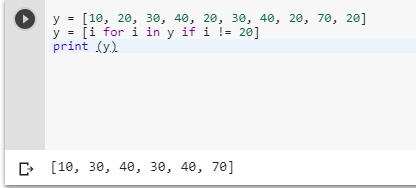# Is there a simple way to delete a list element by value?

1 view

a = [1, 2, 3, 4]

b = a.index(6)

del a[b]

print a

The above shows the following error:

Traceback (most recent call last):

File "D:\zjm_code\a.py", line 6, in <module>

b = a.index(6)

ValueError: list.index(x): x not in list

So I have to do this:

a = [1, 2, 3, 4]

try:

b = a.index(6)

del a[b]

except:

pass

print a

But is there not a simpler way to do this?

by (107k points)

To remove the first occurrence of an element by value in a list, you can simply use list.remove():-

x = ['a', 'b', 'c', 'd', ‘b’]

x.remove('b')

print (x )To remove all the occurrences of an element in a list you can use a list comprehension:-

y = [10, 20, 30, 40, 20, 30, 40, 20, 70, 20]

y = [i for i in y if i != 20]

print (y)